Elite indicators :) - page 163109

Mtf Stoch Histo

mtf

interpolate

thanks

Files:159

Thank you

Perfect. Thank you!

Like this?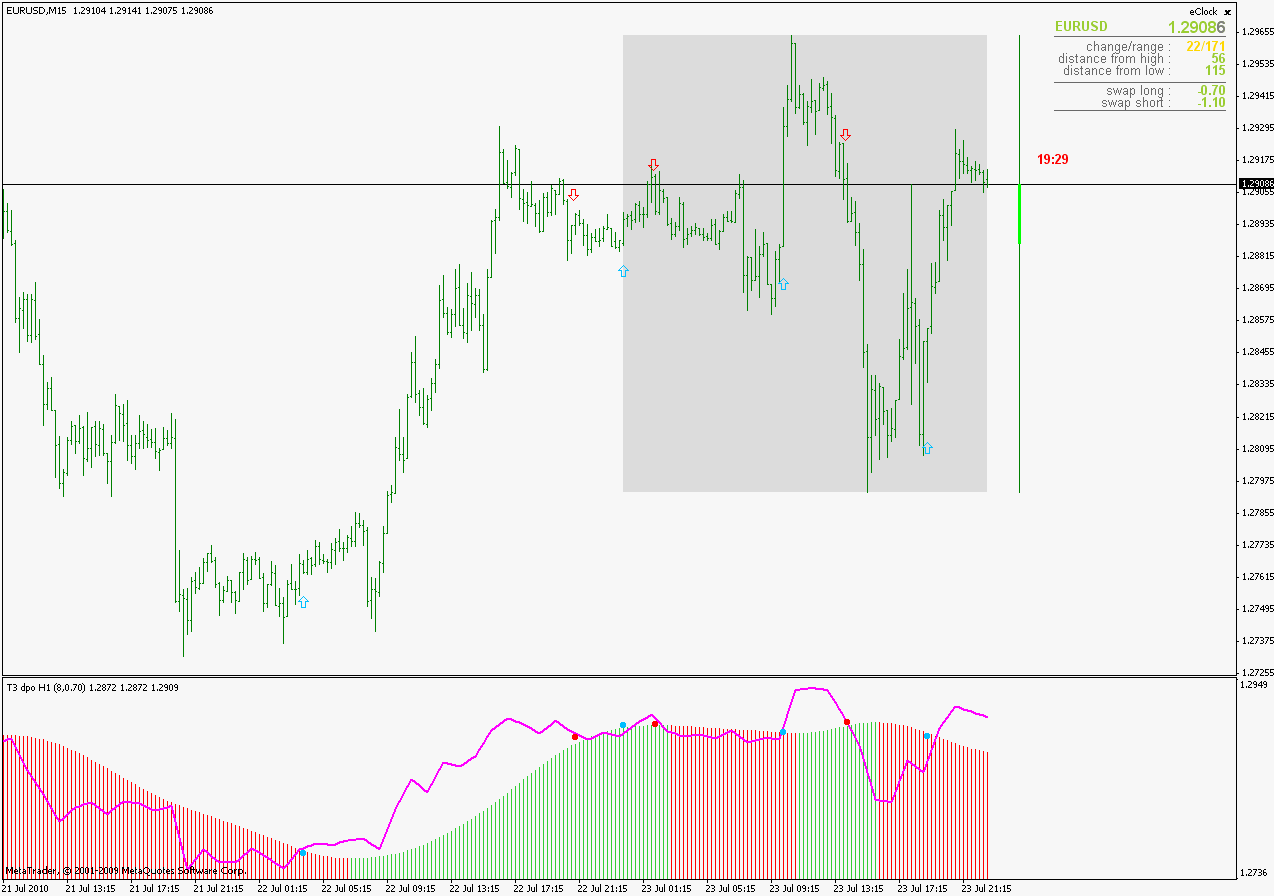regards2970
Hercs

An apology together with gratitute

ValeFX

OK, lets try it outAustin Passamonte have published something very similar 2 years ago, so it is a kind of a "republishing" of that idea. This one is a version where default values for pivot levels are at 38,61% of the range, but you can easily change it through parameters to the fibo levels Passamonte proposes in his TASC article (0.25,0.50 and 0.75, and then it looks like the picture bellow) or whatever levels you want. If level parameter is set to 0 or less than 0, it will be omitted.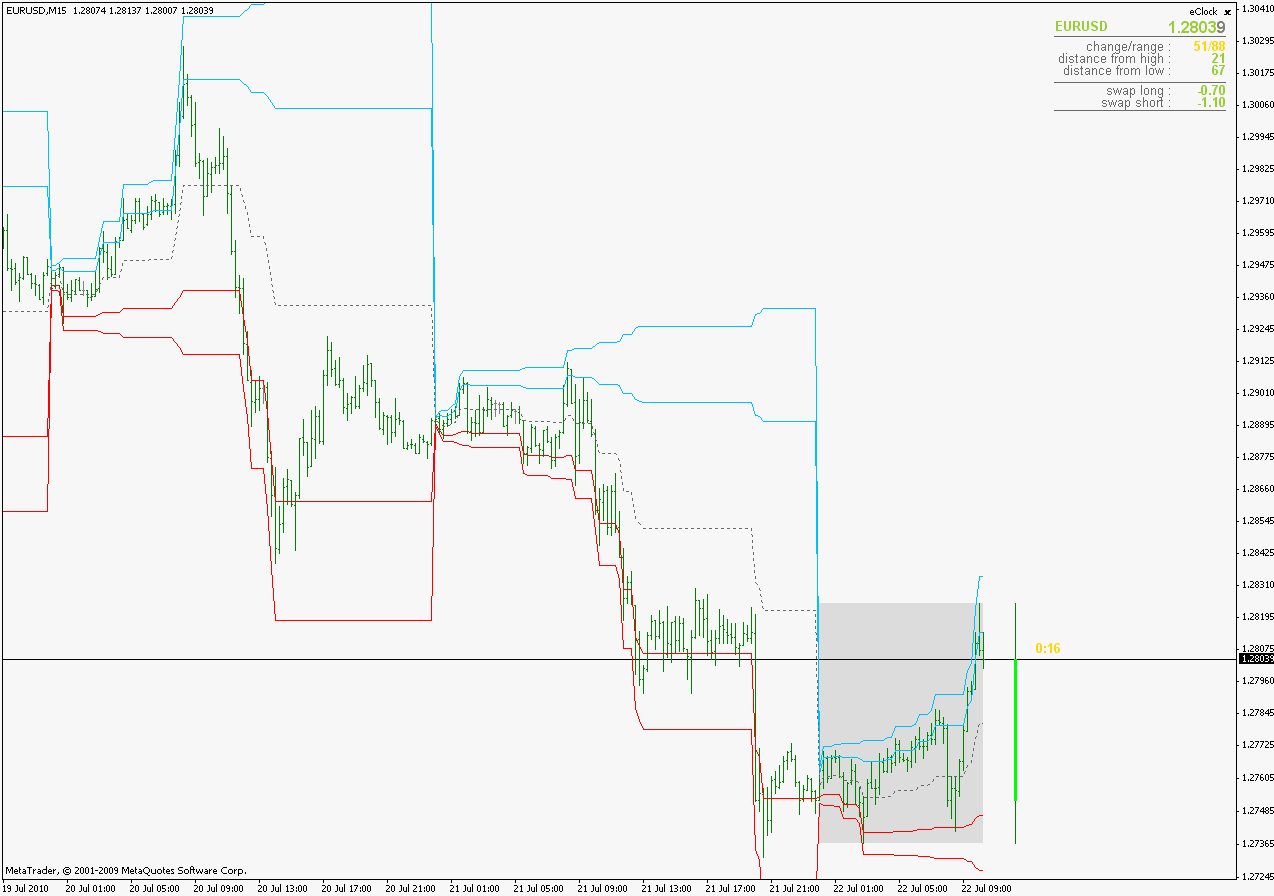PS: Passamonte in his calculations (published here : CM Dynamic Pivots* TradeStation ) uses a bit different calculation (he actually prefers low) but included that calculation way in the indicator as "AlternativeCalculation" and it looks like this (with levels set to 0.38 and 0.61) It, implicitly, includes levels 25 since what he calls "midHigh" and "midLow" are in fact 25% levels. Since it looks better than the 25,50 and 75% calculation, decided to set those as default parameters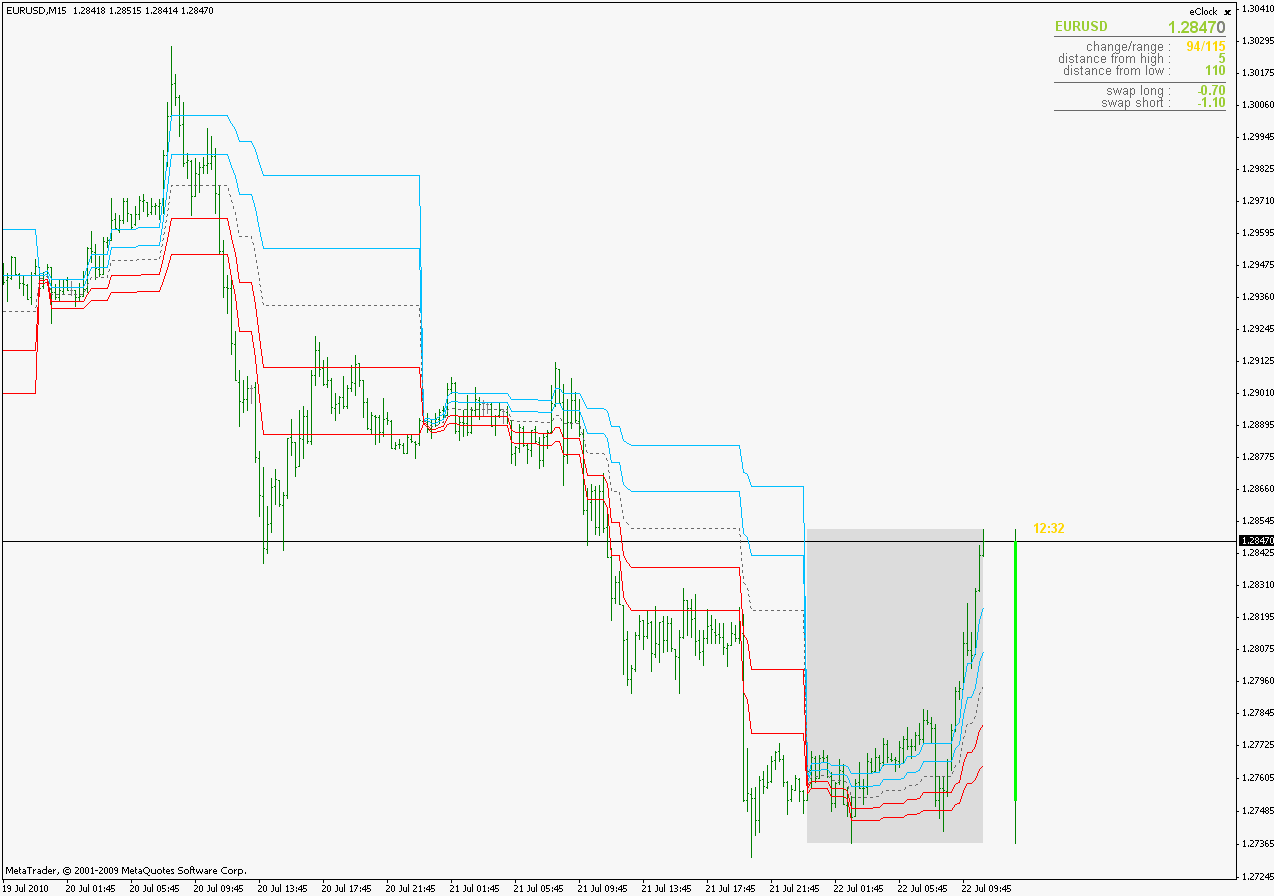============================

Firstly an apology for not having thanked you earlier, but for some strange reason, I missed this post.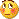Much appreciate you and your time.

I will run it this week and report back my findings.

Sincerely.2970
Hercs
Peter,

Here is one that can do what you asked for

It is a different indicator (as you will find out) Reasons for not adapting the one you posted : it sometimes gives wrong alert (at least it is sometimes giving it to me while I change time frames) So I used one that I have. Color changes are the same if you use ChangeOnDirectionChange (see the comparison - SignalEMA is set to 0 in the lower one and then it does not show signal line)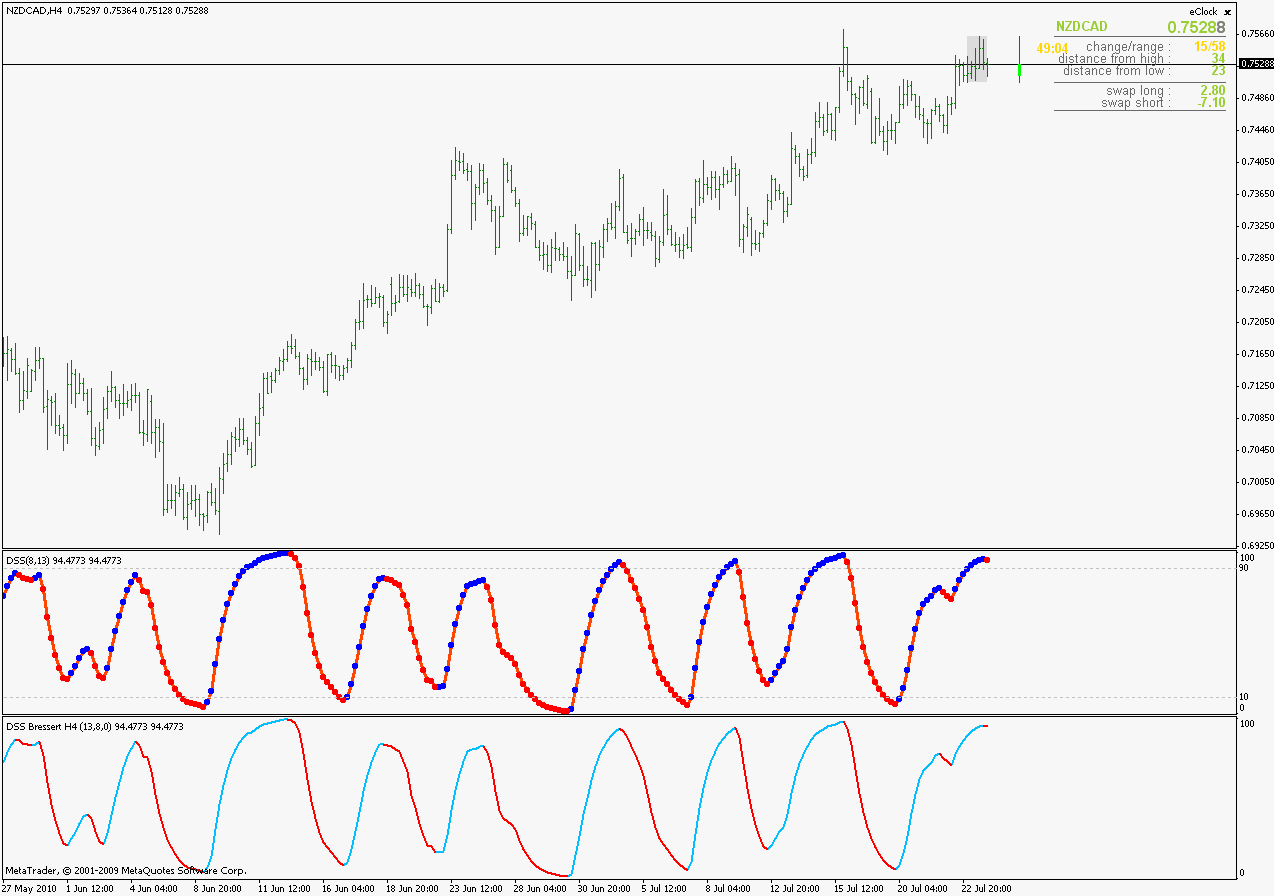About ChangeOnDirectionChangeparameter : this indicator can change colors and the way it alerts depending on this parameter. If it is set to true it will change color (and alert) when slope of dss changes. If it is set to false and SignalEMA is greater than 0, then it will change color (and alert) on dss - signal line crosses (see the compared : upper shows slope change, lower shows signal line crosses)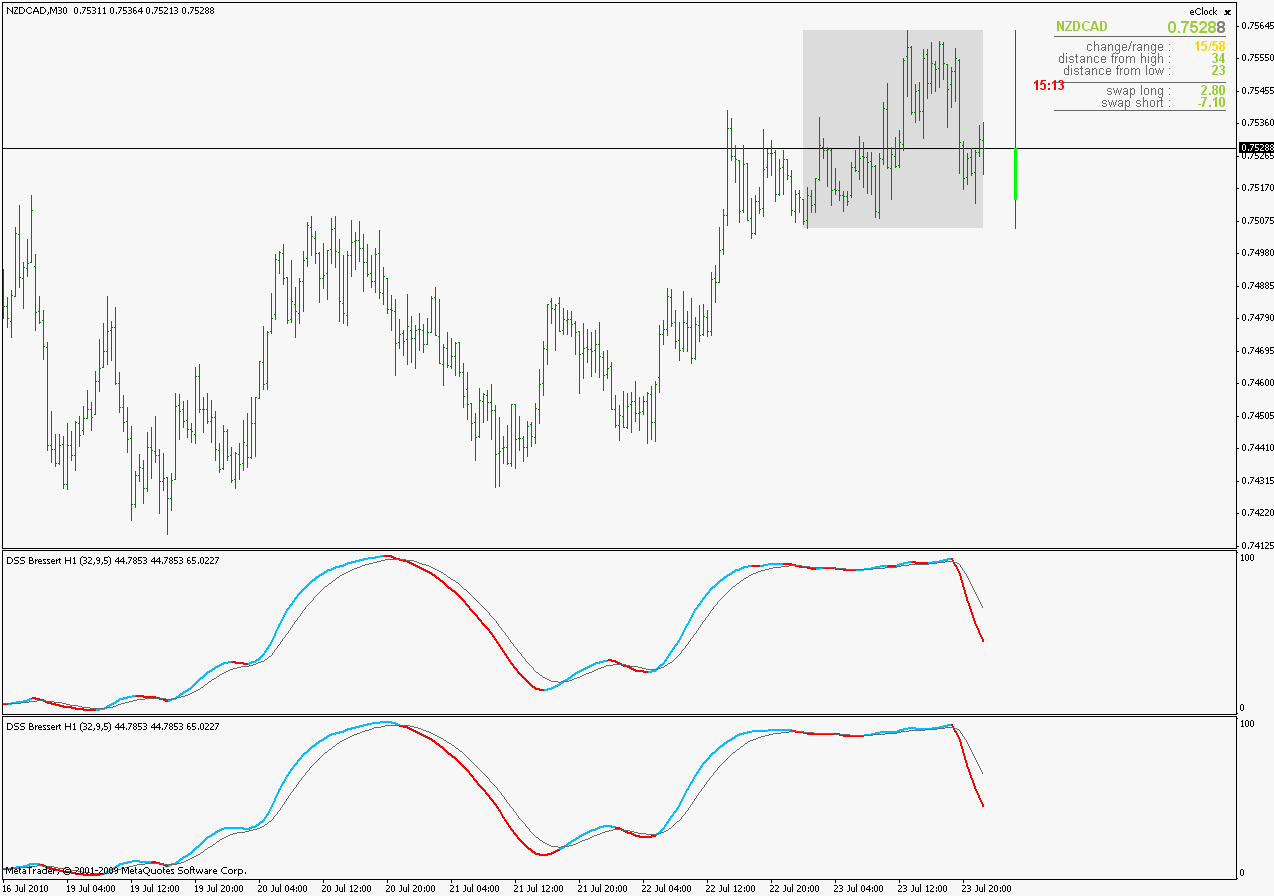Parameter that you need : ShowHigherTimeFrame. If set to true it will automatically show one time frame higher than the current time frame (unless it is monthly, when there is no higher time frame)

____________________________

PS: default lengths in this one are changed from 13,8 to 32,9 hence the difference if you just place it on chart without changing parameters

regards

==============================

Mladen, when you have some time, would you mind adding a "dot"/arrow on the crossing of the indicator, please?

From what I can see, this is a vast (!) improvement over the previous one, which I display here in the first sub-window.

Much appreciated.

Best regards.

Files:54

Bressert

peterke:
Mladen, many tks I will give it go

rgds

Peter

Mladan, working well, I really appreciate your help

regards

Peter76

Hi

Hi Mladan, many thanks arrow type!!30

Attached is the TriangularMA centered abands alerts indicator.

This indicator gives alerts and emails when the price crosses the bands.

Can you modify this indicator to give alerts when:

The previous candle has touched the band and the present candle is of opposite color (Black for upper band and white for lower band).

Thanks

Umesh

Files:2970
Hercs

snaking RSiOMA_v4

Good morning to you Mladen. Trust you had an enjoyable weekend with your family.

I attach here the RSiOMA that you improved on. My request is for a "Interpolate" version please.

Looking forward to hearing from you and thanking you in advance.

Best wishes.

Files:89

Dynamic Balance Point Indicator

I've come across this concept a couple of times recently and it grabbed my attention. Do you or anyone else here have or know of a Fibonacci Dynamic Balanced Point Indicator or a Fixed Balance Point Indicator for MT4?

I found this code for Metastock Indicators written by Adam Hefner, but no explaination.

Dynamic Balanced Point Indicator

dt:=DayOfWeek();

DBC:=(HighestSince(5,DayOfWeek()=dt,H)+

LowestSince(5,DayOfWeek()=dt,L)+CLOSE)/3;

DBC

Fixed Balance Point Indicator

{Fixed Balance Point Calculation}

FBC:=If(Mc1=0 AND Fc1>2,

{then}(Ref(HHV(H,LastValue(mc1)),-1)+

Ref(LLV(L,LastValue(Mc1)),-1)+

Ref(C,-1))/3,

{else}If(Fc1=0 AND Mc1>5,

{then}(HHV(H,LastValue(Fc2))+

LLV(L,LastValue(Fc2))+C)/3,

{else}If(Fc1=0,

{then}(HHV(H,LastValue(Mc1))+

LLV(L,LastValue(Mc1))+C)/3,

{else}0)));

{Fixed Balance Point Plot}

FBP:=ValueWhen(1,FBC>0,FBC);

FBP;

I also found this post recently on FF by a fellow named HiddenGap explaining the concept a bit more thoroughly.

The Dynamic Balance Point Line is based on Drummond Geometry, the work of Robert Krause, and Chaos Theory (Bill Williams).

Basically, we are plotting a DYNAMIC higher timeframe on the chart we are looking at.

I will use a daily chart for the construction here.

The daily balance point= (H+L+C)/3

The weekly balance point = (Highest high of the week + lowest low of the week+friday's close)/3. This can only be calculated at the end of the week.

BUT what if we simulate a week every 5 trading days? In other words, after Tuesday, we look back at the last 5 days and take the highest high in those 5 days and the lowest low in those 5 days plus Tuesday’s close and calculate a simulated weekly close. This simulated weekly close would be dynamic because after each day it would calculate again.

If we were using a real week then it would be calculated on Friday and stay the same until the next Friday. A DYNAMIC week changes every day.

Once we have the dynamic balance point. We create a Wilder's smoothed moving average of 5 and then we plot it forward 3 days.

So the basic formula is:

(HHV(5)+LLV(5)+C)/3=DBP

DBPL= 5 period Wilders smoothed moving average plotted 3 intervals into the future.

So far, I haven't been able to locate an mq4. It looks quite good actually from what I have read....

if you can help me with this I would greatly appreciate it!

Thank you,

fudo

edit: I found one other code sample on another page with this description -

Fibonacci Trader - Dynamic Multiple Time Frame

Description: Dynamic Multiple Time Frame Indicator Explanation of the Dynamic Multiple Time Frame Indicator by the author, Adam Hefner: "The Fixed Balance Point is calculated every Friday by taking the weekly (high+low+close)/3. It really doesn't need to be plotted, but is mostly used to base the other indicators from. The Fixed Balance Point Step, is a 5 week average of the Fixed Balance Point. The Dynamic Balance Point is the daily update of the Fixed Balance Point. The Dynamic Balance Point Step is the daily update of the Fixed BalancePoint Step. Robert Krausz teaches that by watching the balance point calculations of the longer (weekly) time, you have the market direction (trend) for the shorter (daily) time. He also revealed that the when the Dynamic Balance Point is above the Dynamic Balance Point Step, then the trend is up, and oppositeis true for down trend. I have found that these act in much the same way as a 5/25 moving average cross-over system. I like the Fibonacci Support & Resistance best of all, seems that these support/resistance areas are very easy to visualize using this formula."

FT-DBP

dt:=DayOfWeek();

DBC:=(HighestSince(5,DayOfWeek()=dt,H)+

LowestSince(5,DayOfWeek()=dt,L)+CLOSE)/3;

DBC

FT-Weekly Price Range Calculation

WRC:=If(Mc1=0 AND Fc1>2,

{then}Ref(HHV(H,LastValue(mc1)),-1)-

Ref(LLV(L,LastValue(Mc1)),-1),

{else}If(Fc1=0 AND Mc1>5,

{then}HHV(H,LastValue(Fc2))-

LLV(L,LastValue(Fc2)),

{else}If(Fc1=0,

{then}HHV(H,LastValue(Mc1))-

LLV(L,LastValue(Mc1)),

{else}0)));

WRP:=ValueWhen(1,WRC>0,WRC);

{Resistance Range}

RR1:= FmlVar("FT-FBP","FBP")+(WRP*.5);

RR2:= FmlVar("FT-FBP","FBP")+(WRP*.618);

{Support Range}

SR1:= FmlVar("FT-FBP","FBP")-(WRP*.5);

SR2:= FmlVar("FT-FBP","FBP")-(WRP*.618);

{Plot Ranges}

RR1;

RR2;

SR1;

SR2;

FT-Dynamic Balance Point Step

DPS:=(ValueWhen(1,FmlVar("FT-DBP","DBC"),

FmlVar("FT-DBP","DBC"))+

ValueWhen(5,FmlVar("FT-DBP","DBC"),

FmlVar("FT-DBP","DBC"))+

ValueWhen(10,FmlVar("FT-DBP","DBC"),

FmlVar("FT-DBP","DBC"))+

ValueWhen(15,FmlVar("FT-DBP","DBC"),

FmlVar("FT-DBP","DBC"))+

ValueWhen(20,FmlVar("FT-DBP","DBC"),

FmlVar("FT-DBP","DBC")))/5;

DPS;

FT-FBP

{Fixed Balance Point Calculation}

FBC:=If(Mc1=0 AND Fc1>2,

{then}(Ref(HHV(H,LastValue(mc1)),-1)+

Ref(LLV(L,LastValue(Mc1)),-1)+

Ref(C,-1))/3,

{else}If(Fc1=0 AND Mc1>5,

{then}(HHV(H,LastValue(Fc2))+

LLV(L,LastValue(Fc2))+C)/3,

{else}If(Fc1=0,

{then}(HHV(H,LastValue(Mc1))+

LLV(L,LastValue(Mc1))+C)/3,

{else}0)));

{Fixed Balance Point Plot}

FBP:=ValueWhen(1,FBC>0,FBC);

FBP;

FT-Fixed Balance Point Step

FPS:=(ValueWhen(1,FmlVar("FT-FBP","FBC")>0,

FmlVar("FT-FBP","FBC")) +

ValueWhen(2,FmlVar("FT-FBP","FBC")>0,

FmlVar("FT-FBP","FBC")) +

ValueWhen(3,FmlVar("FT-FBP","FBC")>0,

FmlVar("FT-FBP","FBC")) +

ValueWhen(4,FmlVar("FT-FBP","FBC")>0,

FmlVar("FT-FBP","FBC")) +

ValueWhen(5,FmlVar("FT-FBP","FBC")>0,

FmlVar("FT-FBP","FBC")))/5;

FPS151107

casaliss

Here you go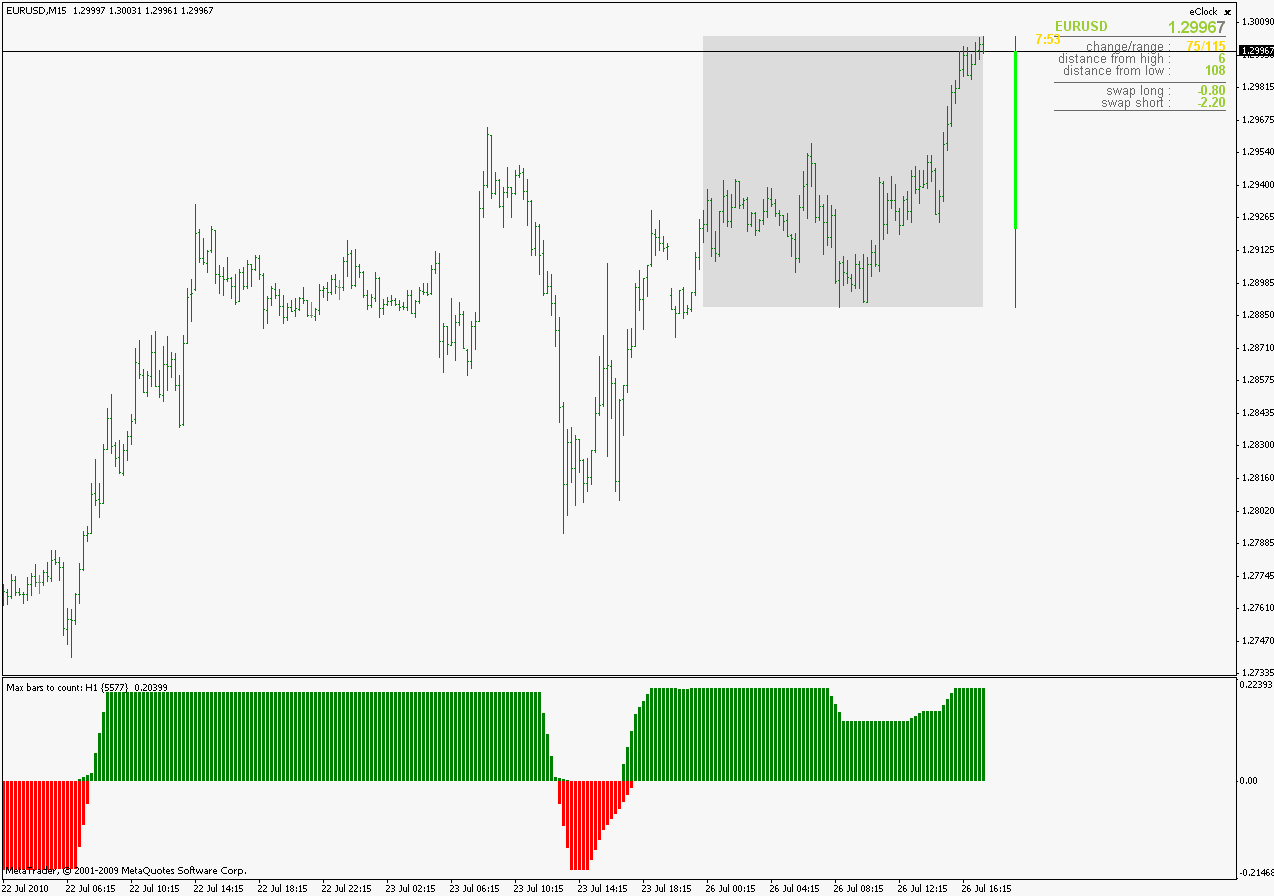regards